# Math Formula

Overview

The Math Formula question allows students to easily enter complex math as a response, with advanced validation capabilities using math specific scoring methods, in conjunction with our purpose built math engine.

Use the Math Formula question type if the student response only requires one line of math. If you need the student to use multiple lines of math, you may want to use Cloze Math or Math Essay.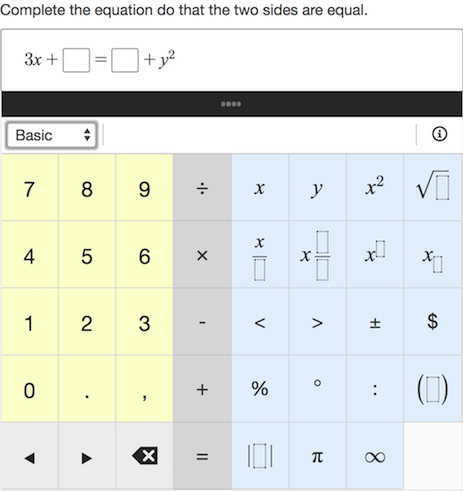Figure 1: Math formula question example.

## Create a question

Enter the question stem in the Compose Question area. If you want to insert some math in the stimulus, you can use the Math Editor. Note that the student will not be able to interact with math entered this way.

To access the Math Editor, click inside the Compose Question text area and select Math Editor from the Rich Text Editor panel (see Figure 2).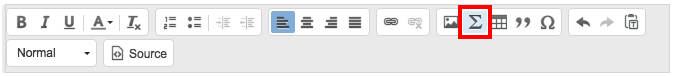Figure 2: Math editor button in the rich text toolbar.

A new window with a keypad will pop up and you will be able to add a LaTeX or MathML expression.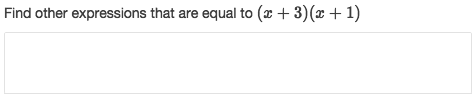Figure 3: Question with math expression in the stimulus.

## Template Field

Student response boxes (and math expressions) are added in the Template area. This is a LaTeX only area, and math expressions and response boxes are entered using the LaTeX Keypad - as seen in Figure 4 below.

It is important to remember that everything entered into the Template area must also be entered into the Value field in the validation area, or the question will not validate properly.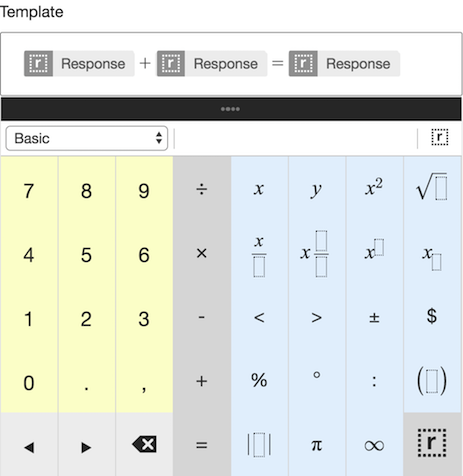Figure 4: Authoring a math question with the LaTeX keypad.

## Validation

Insert a value in the Point(s) box to set the score for the question. The default value is 1.

Underneath the Point(s) area, select a suitable math scoring method from the Method drop down menu. There are various scoring methods available in math question types.

Enter the correct response in the Value field.

Important: anything that has been entered by the author in the Template field below the Compose Question field, must be entered in the validation Value field. If the Template field and the validation Value field do not match, it will not be possible for the student to enter a correct response.

In the case below, the correct response must be in the form x + y = z, and the sum of two terms must be 3/4. It is only necessary to enter one solution in the Value field.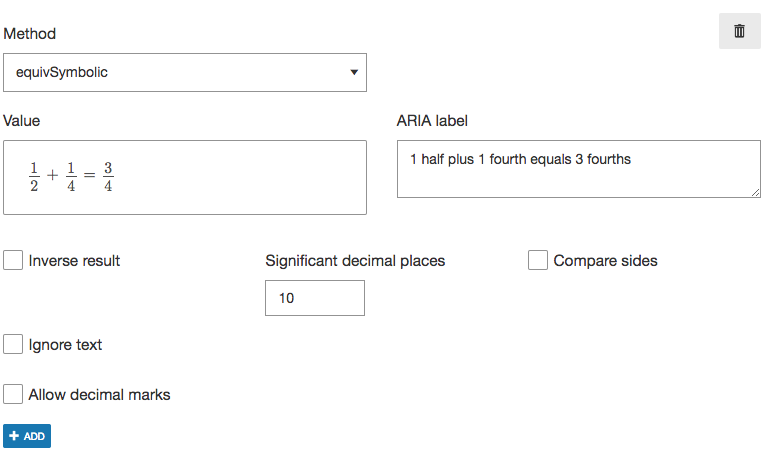Figure 5: Setting a value. The entire equation entered into the LaTeX template area must be entered into the value area.

Each scoring method has additional options that allows authors to set more precise validation conditions. When you select a scoring method, all of its extra options will be displayed underneath the Value field. Details of additional options can be found on the articles for each scoring method.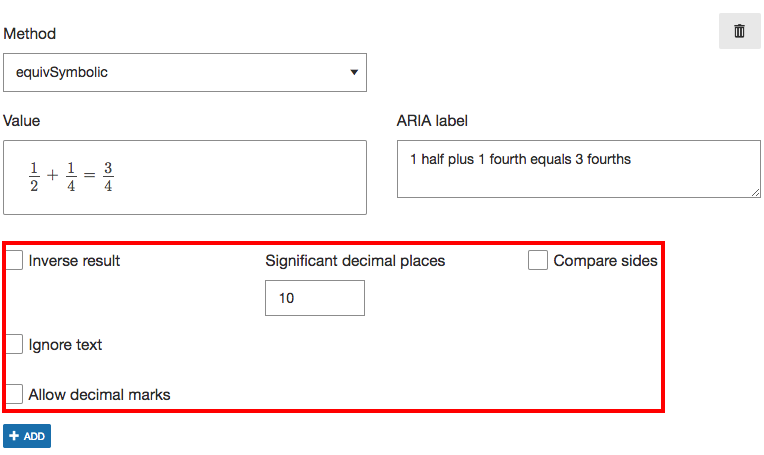Figure 6: Additional options.

## More Options: Scoring

• Unscored/Practice usage - Removes all scoring from the question.
• Penalty point(s) - The value entered here will be deducted from the student for an incorrect answer.
• Minimum score if attempted - Set attempt marks for the question, which will be awarded to the student even if their response is incorrect.
• Check answer button - Show or hide the check answer button from the student. The check answer button allows students to check if their answer is correct/incorrect. Clicking this button does not tell the student what the correct answer is.
• Check answer attempts - The value entered here refers to how many times the student can use the Check answer button, before it is automatically disabled.

## Layout

• Transparent background - If this is enabled, the background of the response area will match the color of the page background.
• Response minimum width (px) - Enter, in pixels, the minimum width of the response area.
• Template font scale - This is referring to the content in the Template field only. It is set to Boosted by default, so it will appear slightly larger. You can change it to Normal, if you would like it to match the size of the rest of the question text.
• Response containers (global) and Response containers (individual) - Configure the Width and the Height, in pixels, for either all response containers (global), or for specified response containers (individual).